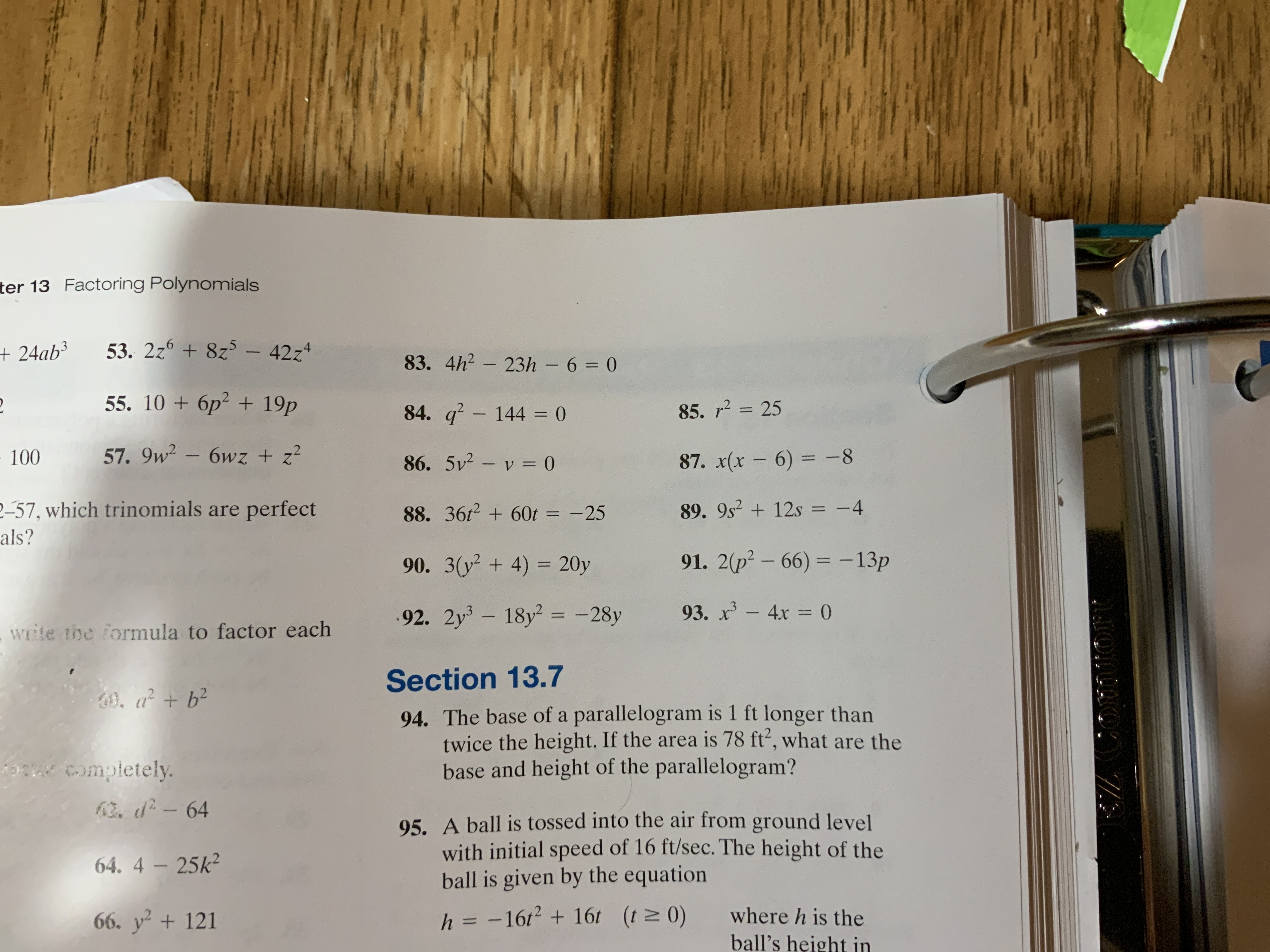# ter 13 Factoring Polynomials53. 2z6 8z5 - 42z4+24ab383. 4h2-23h- 6= 055. 10 + бр? + 19p85. 2584. q - 144 = 057. 9w2 - 6wz + z210086. 5v2 - v 087. x(x - 6) = -8P-57, which trinomials are perfect89. 9s212s = -488. 367260t =-25als?91. 2(p2 - 66) - 13p90. 3(y2 4) 20y193. x-4x = 0.92. 2y3-18y2= -28ywote the formula to factor eachSection 13.7a + b294. The base of a parallelogram is 1 ft longer thantwice the height. If the area is 78 ft', what are thebase and height of the parallelogram?completely62. d-6495. A ball is tossed into the air from ground levelwith initial speed of 16 ft/sec. The height of theball is given by the equation64. 4-25k2where h is theh = -162 + 16t (t 0)66. y2 +121ball's height in

Question
18 viewshelp_outlineImage Transcriptioncloseter 13 Factoring Polynomials 53. 2z6 8z5 - 42z4 +24ab3 83. 4h2-23h - 6 = 0 55. 10 + бр? + 19p 85. 25 84. q - 144 = 0 57. 9w2 - 6wz + z 2 100 86. 5v2 - v 0 87. x(x - 6) = -8 P-57, which trinomials are perfect 89. 9s212s = -4 88. 367260t =-25 als? 91. 2(p2 - 66) - 13p 90. 3(y2 4) 20y 1 93. x-4x = 0 .92. 2y3-18y2= -28y wote the formula to factor each Section 13.7 a + b2 94. The base of a parallelogram is 1 ft longer than twice the height. If the area is 78 ft', what are the base and height of the parallelogram? completely 62. d-64 95. A ball is tossed into the air from ground level with initial speed of 16 ft/sec. The height of the ball is given by the equation 64. 4-25k2 where h is the h = -162 + 16t (t 0) 66. y2 +121 ball's height in fullscreen
check_circle

Step 1

Given:

Step 2

### Want to see the full answer?

See Solution

#### Want to see this answer and more?

Solutions are written by subject experts who are available 24/7. Questions are typically answered within 1 hour.*

See Solution
*Response times may vary by subject and question.
Tagged in

### Polynomials